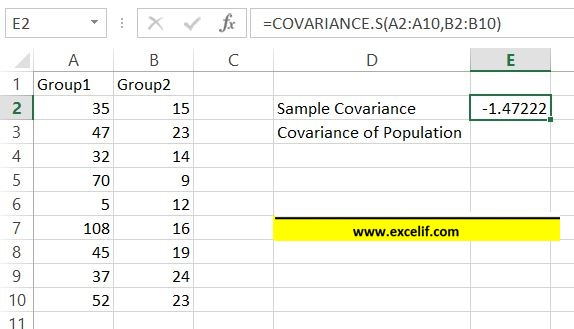How To Calculate Covariance In Excel?

In this Excel tutorial you will teach yourself how to calculate covariance in Excel.

We can detect the existence of a correlation relationship by examining the covariance.

Covariance is the average of the products of the deviations of each data point pair.

Use covariance to define the relationship between two datasets.

• A positive value of covariance occurs when both examined characteristics are moving in the same direction.
• Negative covariance occurs when the increase in the value of one feature tends to decrease the value of the other.

## Sample Covariance

To calculate sample covariance use COVARIANCE.S Excel function.

COVARIANCE.S syntax:

=COVARIANCE.S(array1,array2)

In the given example sample covariance formula is

=COVARIANCE.S(A2:A10,B2:B10)## Population Covariance

To calculate population covariance use COVARIANCE.P Excel function.

COVARIANCE.P syntax:

=COVARIANCE.S(array1,array2)

In the given example sample covariance formula is =COVARIANCE.P(A2:A10,B2:B10)In most cases you will use sample covariance. Use covariance of population function only when it is specifically said that it population covariance.

## Template

```Further reading: